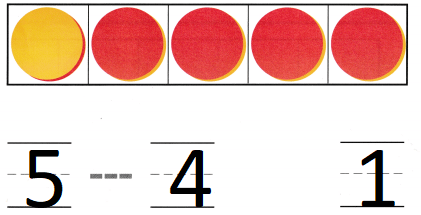# Texas Go Math Kindergarten Module 9 Assessment Answer Key

Refer to our Texas Go Math Kindergarten Answer Key Pdf to score good marks in the exams. Test yourself by practicing the problems from Texas Go Math Kindergarten Module 9 Assessment Answer Key.

## Texas Go Math Kindergarten Module 9 Assessment Answer Key

Concepts and Skills

DIRECTIONS: 1. There are 3 counters. Write the number of yellow counters. Write the number of red counters. (TEKS K.2.I) 2. Write the number of counters in all. Write the number of yellow counters. Write the number of red counters. (TEKS K.2.I)

Question 1.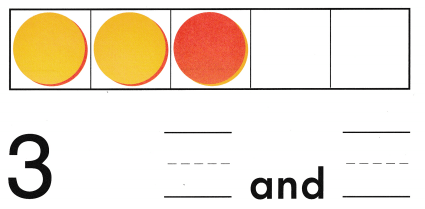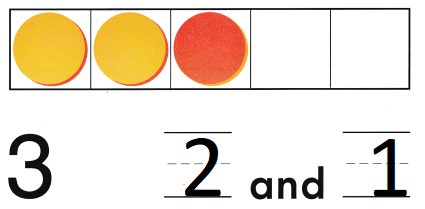Explanation:
The number of red counters are 1
the number of yellow counters are 2
Total number of counters 3
2 + 1 = 3

Question 2.Explanation:
The number of red counters are 2
the number of yellow counters are 2
Total number of counters 4
4 = 2 + 2

DIRECTIONS: 3. Count the counters in the five frames. Write the number of red counters. Trace the symbol. Write the number of yellow counters. (TEKS K.2.I) 4. Leila has 5 counters. She has 4 red counters. The rest are yellow. How many yellow counters does Lelia have? Write the numbers and trace the symbol. (TEKS K.2.I)

Question 3.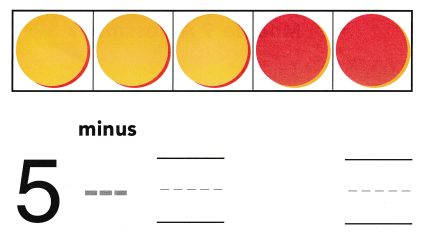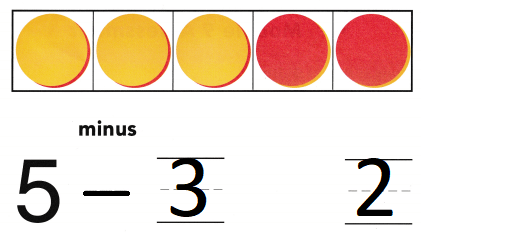Explanation:
The number of red counters are 2
the number of yellow counters are 3
Total number of counters 5
5 – 3 = 2

Question 4.
Texas Test Prep